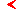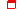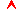﻿ Dynamic systems and bifurcations - Program of course - Education - Saratov group of theoretical nonlinear dynamicsmainthis page only

# Dynamic systems and bifurcations

The author: Doctor of Sciences, Professor Kuznetsov A. P.

17 lectureseducationproblems and lessons

## Section 1. Dynamic system. The basic definitions, examples and properties.

1.1 About classification of dynamic systems. Flow systems and mappings. The dissipative and conservative systems, character of evolution of a cloud of points in a phase space. Attractors. Dimensionality of a phase space, growth of variety and complexity of attractors with increasing number of dimensions. The elementary examples of discrete mappings: a chain of resistances and a biological population. A method of sections of Poincare. About interrelation of streams and mappings. Invertible and noninvertible mappings. Autonomous and nonautonomous dynamic systems. Poincare section for nonautonomous systems.

1.2 Examples of dynamic systems. Why should we consider dynamic systems in nonlinear dynamics? The kinetic equations and the law of corresponding states. Brusselator. Oscillatory chemical reactions. Oregonator. Rosler system as a an artificial model of a chemical kinetics. Fermi acceleration and problems with collisions. Ulam model. Zaslavski and Chirikov system (springing ball on a vibrating table), approximate discrete mapping for the case of a small vibration amplitude, its dissipativity and invertable. System with viscous friction under impuls forcing, Henon-type maps and Henon map, their dissipativity and invertibility. The logistic mapping, cubic mapping, their noninvertibility. A periodically forced relaxation generator. Circle map, representation of its dynamics on the iterative diagram. When this mapping is noninvertible?

## Section 2. The elementary bifurcations of flows

2.1 Bifurcations of the one-dimensional flow systems. Links between the theory of bifurcations and theory of catastrophes in case of the one-dimensional systems. Classification of bifurcations by a codimension. A saddle - node bifurcation. Transcritical bifurcation. A "fork" bifurcation. Interconnections of various bifurcation diagrams at the two-parameter analysis. Subcritical and supercritical types of bifurcations.

2.2 Bifurcations of the one-dimensional flows in two-dimensional systems. Nontriviality of a problem (after Prigogine). A conservative oscillator near the points of catastrophes. Dependence of frequency on parameters and a phenomenon of a softening of modes. Physical examples. Power laws near the points of catastrophes. A case of the dissipative oscillator. Transmutations of focuses into nodes near the points of catastrophes or why the "saddle-focus" bifurcation is not possible. Deceleration of time and universality of bifurcations. Phase portraits of bifurcations of the one-dimensional flows in two-dimensional systems.

2.3 Andronov-Hopf bifurcation. About a possibility of bifurcations of the dynamic systems which do not have analogs in the theory of catastrophes. Andronov-Hopf bifurcation and its universality. Evolution of points in a three-dimensional phase space at a threshold of Andronov-Hopf bifurcation and concept of central manifold. The "linear part" of the problem and its reduction to a canonical form in a framework of the slow amplitudes formalism. A role of nonlinearity and the universal equations for slow amplitudes. Configuration of the born limit cycle in canonical variables and in an initial phase space. A threshold of Andronov-Hopf bifurcation in two-dimensional systems. A threshold of Andronov-Hopf bifurcation in three-dimensional systems.

2.4 Other bifurcations of bidimentional streams. A bifurcation of birth of a limit cycle from an inspissation of phase trajectories and its explanations in terms of slow amplitudes. Nonlocal bifurcations of a codimension one: occurrence and decay of the saddle bundle, birth of a limit cycle from a loop of a separatrix, birth of a limit cycle from a common separatrix of a saddle and a node. Bifurcations of a codimension two. "Critical point" of a line of Andronov-Hopf bifurcation (transmutation of a line of a subcritical bifurcation into supercritical) and its connection with a bifurcation of birth of a limit cycle from an inspissation of phase trajectories. Cusp of bifurcation lines of birth of a limit cycle from an inspissation of trajectories and analog of a "fork" bifurcation for cycles. A bifurcation "a common point of Andronov-Hopf bifurcations and a saddle - node", its relationship with a birth of a limit cycle from a loop of a separatrix.

2.5 From two-dimensional flows - to one-dimensional maps. A method of sections of Poincare for two-dimensional flows. Various examples of phase portraits and the relevant iterative diagrams. A problem of reconstruction of the one-dimensional mapping. Invertibility of mappings associated with two-dimensional flows.

## Section 3. Mappings and their bifurcations

3.1 The elementary properties of the one-dimensional mappings. The fixed points. Stable and unstable fixed points. Character of convergence (divergence) near the fixed point. A multiplicator and its geometrical interpretation. Stability criterion for the fixed point. Examples of iterative diagrams for different values of a multiplicator. Cycles. Relationship between a problem of searching a N-cycle and a problem of searching the fixed point. A multiplicator of a cycle and its expression as product of derivatives. Examples of evaluation of a multiplicator of a cycle. Concept of superstable cycles. About variety of cycles by an example of the logistic mapping.

3.2 Bifurcations of a codimension one. The tangential bifurcation. Motion of the imaging point inside a "corridor" near bifurcation threshold, description of a bifurcation with the help of the differential equation and an estimate of time of passage of "corridor". A bifurcation of a "fork" type. Subcritical types of the tangential bifurcation and a "fork". Bifurcation. Period doubling bifurcation. Birth of a 2-cycle in the logistic mapping and analysis of a stability of this cycle. Period doublings " in terms " of a twice iterated mappings. Properties of such mappings and Schwarz derivative. A bifurcation " the hard transition through a multiplicator -1 " as alternative to period doubling. Bifurcations of cycles. From local to the global bifurcation analysis: period doubling cascade, a bifurcation tree for the logistic mapping and discussion of its structure.

3.3 Bifurcations of a codimension two. A cusp point of the tangential bifurcations. A point of transmutation of a line of period doubling into a line of the hard transition (subcritical period doubling) - a flip bifurcation of a codimension two. The stable configurations of stability domains of cycles on a plane of parameters, "crossroad area" and "spring area" situations. Multistability and "multisheet" structure of a parameter plane of multimodal mappings. From local to the global two-parameter bifurcation analysis: examples of coexistence of bifurcation lines and points of various cycles on a parameter plane. Dynamic regimes charts.

3.4 Concept of critical phenomena. Why period doubling bifurcations form a cascade? Accumulation of period doublings. A critical point. Feigenbaum's law and universal constant. Splitting laws for a bifurcation tree and second universal Feigenbaum's constant. Scaling. Scaling along the axis of driving parameter. Scaling on a bifurcation tree. Period doublings in two-parameter mappings. Bifurcation "scenarios" and routes in the parameter plane. A situation of a codimension one associated with a requirement of an "extremum onto extremum" mapping by an example of cubic map. The proof of presence of an extremum of the fourth order for a twice iterated mappings. Tricritical points and the arrangement of the charts of dynamic regimes in their neighbourhood. Two-parameter scaling.

3.5 Two-dimensional mappings. The fixed points of two-dimensional mappings. Monodromy matrix and multiplicators. Examples of various types of dynamics on a phase plane in a vicinity of the fixed point, its relationship with values of multiplicators. Cases of the real and complex multiplicators. Stability of the fixed point. Boundaries of stability domains and domains of the real and complex multiplicators on a plane "trace - Jacobian" of a monodromy matrix. Cycles of two-dimensional mappings. Monodromy matrix and multiplicators of cycles.

3.6 Bifurcations, featured for the one-dimensional mappings in case of two dimensions. Mappings with constant Jacobian, restrictions on bifurcations for such mappings. The tangential bifurcation in Henon maping. Period doubling bifurcation of the fixed point of Henon mapping. Evolution of multiplicators on a complex plane when the parameter is changed from a point of the tangential bifurcation up to a period doubling bifurcation. 2-cycle of Henon mapping and the analysis of its stability.

3.7 Neimark bifurcation. Model mapping in case of the arbitrary Jacobian. Dynamic regimes chart for a model mapping. Attractors as invariant curves and cycles, evolution of imaging point on these attractors. Synchronization tongues. A rotation number. Rational and irrational rotation numbers. Examples of various rotation numbers for the same cycle configuration. Relationship between the rotation number and argument of a complex multiplicator on the line J=1. Stable and unstable manifolds of two-dimensional mappings. Configuration of invariant manifolds for a cycle inside synchronization tongue. Boundary of synchronization tongue as the tangential bifurcation. Fine structure of a plane of parameters in a neighbourhood of a line of an invariant curve. Notes on physical realization of the regimes corresponding to an invariant curve.

3.8 Synchronization and circle mapping. A qualitative explanation of transition to circle mapping while arguing a problem of synchronization. Dynamic regimes chart for a circle mapping. A rotation number for circle mapping. Examples of iterative diagrams for various rational rotation numbers. How does the iterative diagram vary at an exit through boundaries of synchronization tongue? The equation for searching cycle elements and boundaries of synchronization tongue for the given rotation number. Boundaries of the basic tongue.

## Section 4. Three-dimensional flows

4.1 From two-dimensional mappings to three-dimensional flows. Various types of stability of limit cycles in three-dimensional space and their explanation with the help of Poincare sections. Examples.

4.2 The elementary bifurcations of three-dimensional flows. Period doubling bifurcation of limit cycles. A cycle of a "doubled" period as edge of a Mobius strip. Period doubling cascades in a three-dimensional phase space. A bifurcation of birth of a torus and its relationship with Neimark bifurcation. Two time scales, motion at a torus, beats. Ergodic tori and quasiperiodic regimes. Resonant cycles at torus, rational rotation numbers for three-dimensional systems and their relationship with a rotation number in Poincare section. Motion at a torus and on a resonant cycle in a projection onto coordinate plane in a phase space. Tori on the basis of cycles of a doubled period, bifurcation manifolds of tori and the related cycles.

## Recommended literature

1. P.Berge, I.Pomo, K.Vidal. The order in chaos. Moscow: Mir, 1991.
2. Mun F. Chaotic oscillations. Moscow: Mir, 1990.
3. V.S.Anishchenko. The complex oscillations in simple systems. Moscow: Nauka, 1990.
4. M.I.Rabinovich, D.I.Trubetskov. Introduction in a theory of oscillation and waves. Moscow: Nauka, 1984.
5. G.Nicolis., I.Prigogine. Exploring complexity. W.H.Freeman and Company: New-York, 1989.
6. N.V.Butenin, J.I.Neimark, N.A.Fufaev. Introduction to the theory of nonlinear oscillations. Nauka, 1976.
7. T.Poston, I.Stewart. The theory of catastrophes and its applications. Pitman: London, 1978
8. G.Shuster. The deterministic chaos. Physic-Verlag: Weinheim, 1984.
9. Postnov D.E. Bifurcations of the regular attractors. Saratov: "College", 1996.
10. Svirzhev J.M. Nonlinear waves, the dissipative structures and catastrophes in ecology. Moscow: Nauka, 1987.
11. Kuznetsov A.P., Kuznetsov S.P. Critical dynamics of the one-dimensional mappings. Part I. Feigenbaum scenario.//Applied nonlinear dynamics. 1993. V. 1. N 1-2, pp. 15-33.
12. Kuznetsov A.P., Kuznetsov S.P., Sataev I.R. Critical dynamics of the one-dimensional mappings. Part 2. Two-parameter transition to chaos.//Applied nonlinear dynamics. 1993. N 3-4, pp. 17-35.maineducationthis page onlytop

Saratov group
of theoretical nonlinear
dynamics